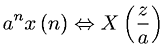Equations > Signal Processing > Z Transform Properties > Z-transform multiplication by an exponential (z domain scaling) property

### Z-transform multiplication by an exponential (z domain scaling) propertyLatex Code:

MathML Code:

 ${a}^{n}x\left(n\right)⇔X\left(\fracza\right)$

MathType 5.0: